#####Bjorn Sjodin March 19, 2013

For a transient simulation, imagine if you could simply insert a virtual sensor in a model at a certain location and then monitor the evolution of a field value over time while solving. In COMSOL Multiphysics you can do just that by using Probes. You define a probe in the Model Builder tree right under the Model Definitions node. Measuring the value at a point is not the only thing you can do with probes, but in this blog post we will focus on that specific task of probing. We’ll also show you how you can use probes to compress the size of a saved transient model by only storing the full solution data set at a few points in time and at the same time having a densely sampled probe data plot with an accompanying table.

### Probing a Fuel Cell Bipolar Plate Simulation

To better illustrate probing, let’s use an example. We’ll show you how to use probes in the case of a transient thermal stress analysis. (Tip: Read our previous blog post for some background on thermal stress analysis.)

Let’s analyze a bipolar plate of a proton exchange membrane fuel cell (PEMFC). The geometry of this model is taken from the Model Library of the Structural Mechanics Module. The PEMFC is a candidate technology for future hydrogen-powered vehicles, but the very expensive materials needed have so far limited its use to high-cost applications, such as certain space missions. Fuel cell simulations contribute to designing less expensive fuel cells and making this technology more widely available. Fuel cell design requires extensive multiphysics simulations including stress, heat, CFD, and electrochemical analysis. We won’t look at a CFD and electrochemical analysis here, but instead show you a mechanical simulation. If you are interested in more advanced fuel cell simulations, check out the model tutorials of the Batteries & Fuel Cell Module.

### About the Fuel Cell Bipolar Plate Model

A fuel cell stack consists of unit cells with an anode, a membrane, and a cathode connected in a series through bipolar plates. The bipolar plates also serve as gas distributors for hydrogen and air that is fed to the anode and cathode compartments, respectively. The picture below shows a schematic drawing of a fuel cell stack. The unit cell and the surrounding bipolar plates are shown in detail in the upper-right corner of the figure.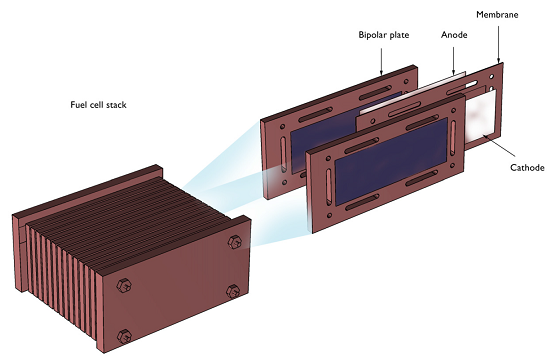The fuel cell operates at temperatures just below 100°C (212°F), which means that it has to be heated at start-up. The heating process induces thermal stresses in the bipolar plates. The figure below shows the detailed model geometry. The plate consists of gas slits that form the gas channels in the cell, holes for the tie rods that keep the stack together, and the heating elements (in the picture labeled Heat source), which are positioned in the middle of the gas feeding channel for the electrodes.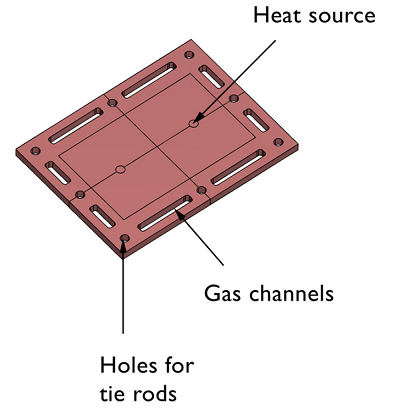In the COMSOL model of the bipolar plate, we use the Thermal Stress user interface and apply a volume heat source to the two central cylindrical domains.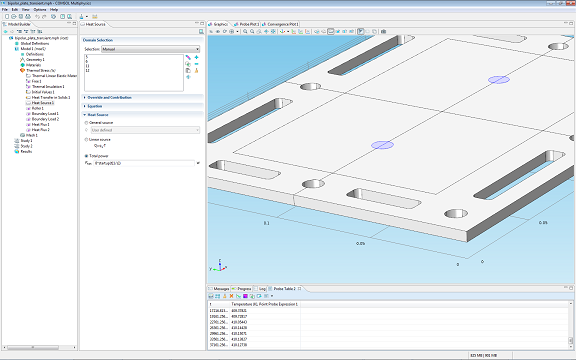There are also convective cooling boundary conditions applied to the sides of the plate.

A total power of 8 W is distributed over the two heating elements. We’ll ramp up the heat power by defining a look-up table that we name startup(), with time as input argument. The bracket notation with 1/s (see image below) converts the input argument t to a unitless quantity before being passed to the look-up table. This unit conversion is not required but it is a good practice to take charge over the unit handling.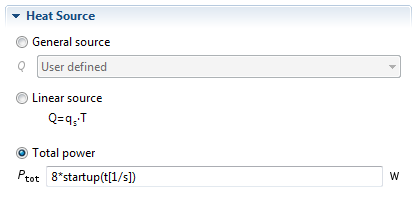The interpolation table is defined under Model Definitions and represents an increase from 0 to 1 over 10 seconds. We use the Piecewise cubic interpolation option to make sure we get a smooth curve. This curve will modulate the input power of 8 W over time so that at t=0 s the input power is 0 W and at t=10 s the input power is the final 8 W. We’ll see below that the time for the bipolar plate to reach steady-state is much longer than 10 seconds due to the relatively inefficient cooling: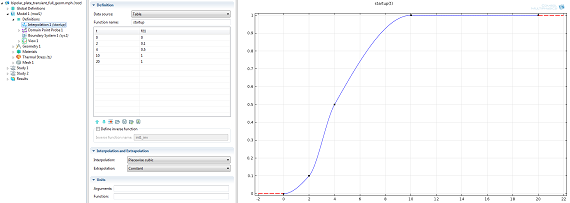### How to Set up Probes in COMSOL Multiphysics

Setting up probes is done from the Model Definitions node in the Model Tree of the COMSOL Desktop. In this case we’ll choose a Domain Point Probe (there are several other types of probes). By using the Point and surface normal Line entry method we get to interactively pick a point on a line that goes from another point on the model boundary with direction normal to the surface of the boundary. The depth can very easily be fine-tuned by the slider control. You also get the exact position of the point in x, y, and z coordinates.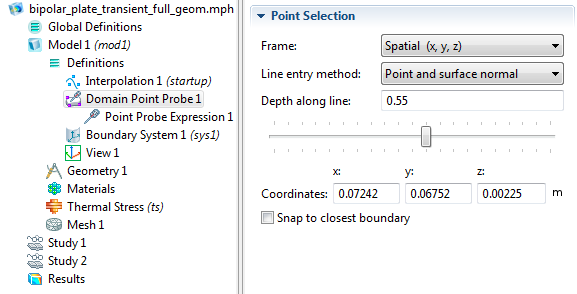The probe location is indicated with a red dot along the line. Here, an arbitrary point is picked on the inside of the plate: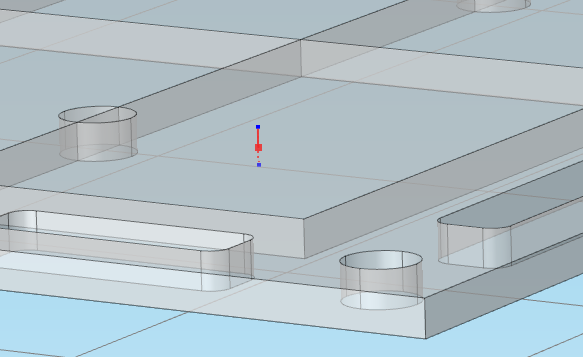Underneath the Domain Point Probe node in the Model Builder tree you will find a node called Point Probe Expression. Here you can set the field variable you wish to evaluate for and monitor during the solution process. The default for thermal stress analysis is the temperature field T but this could really be any field expression you’d like to monitor, including gradient (partial derivative) components. For example, the partial derivative of T with respect to X would be entered as d(T,X) or simply TX (notice the capital X).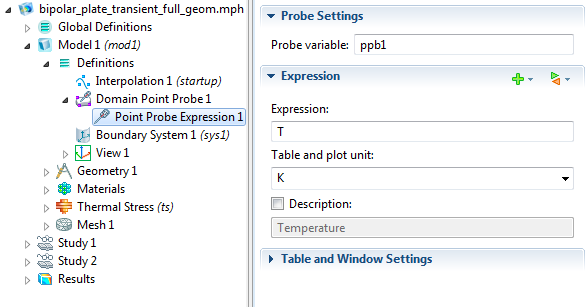In the Study Settings window, we can use the Range tool to specify a start and stop time. Units can be used here and we’ll solve for 10 hours, entered as 10[h].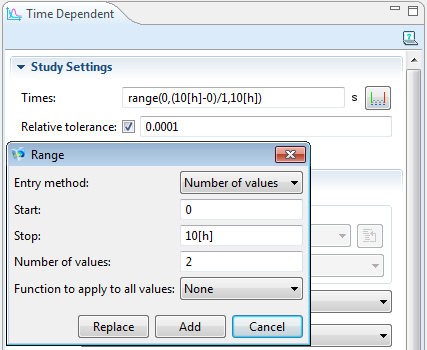### Avoid Storing the Entire Solution with Probing

Now let’s see how probes can be used to avoid storing the entire solution for a large number of time steps. In the Range tool we set the Number of values to 2. This is the smallest possible value and will make sure to only store the full solution at t=0 and t=10[h]. The accuracy of the time-dependent solver is controlled by the Relative tolerance. In this case, the tolerance is lowered from the original 0.01 to 0.0001 (see image above). The lower the tolerance, the shorter the time-steps taken by the solver. This will have an impact on our probe data. We can set the probe to be updated at the same time steps as taken internally by the solver. (The time-stepping algorithm used by COMSOL for this simulation is a so-called variable-order BDF method that adapts its steps in time, based on the solution and the tolerance settings.)

In the Study Settings window, in the section called Results While Solving, we change from the Output from solver option (which would just give us the results at t=0 and t=10[h]) to Steps taken by solver. Like this: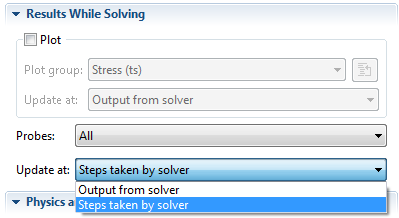Select Compute from the Study node. During the solution, we’ll now see a Probe Plot and Probe Table of the Temperature while the solver is working. If you have a simulation that takes a long time to solve, you can use the displayed Probe information to check if you set something up wrong in the model. Doing so allows you to then stop the simulation before it’s finished, go back and change some settings, and start over again.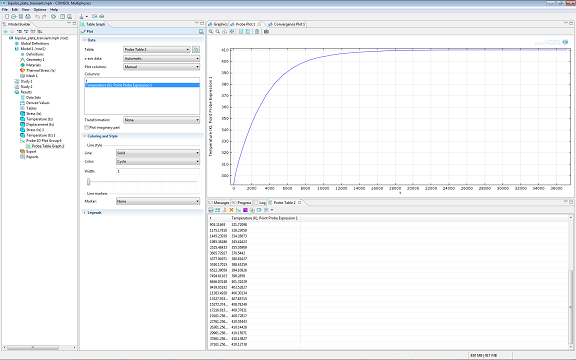As you can see, the probe output has many more data points in time than just t=0 and t=10[h]. By lowering the solver tolerance setting you can increase the number of data points. The table allows you to copy-and-paste the results for use in a spreadsheet. You can also change the settings of the Probe Plot if you wish to use different units or ordering of the axes (here we get temperature T vs. time t).

### Visualizing our Results

As a next step we can of course also visualize the Temperature field at 10 hours (36,000 s) as well as the von Mises Stress with an overlaid mesh plot.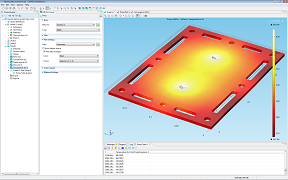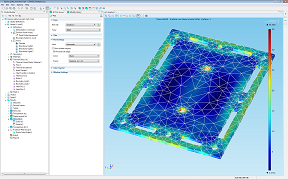Visualization of Temperature field at 10 hours. Visualization of von Mises Stress with overlaid mesh.

Note: The mesh in this example has prismatic finite elements; triangles swept through the thickness of the plate.

#### Post Tags

Technical Content

Using Point Cloud Data in Your COMSOL Model

#### Older Post

Modeling Chemical Reactions: Thermal Stress Analysis

1. Mark Murphy March 21, 2013   3:04 am

Can I get a copy of this as a pdf file?

2. Tina Maake March 1, 2017   1:25 am

I run a Simulation over 16 days, but the multiprobe-table does not Show all values. The first values and time-steps are missing. When I Export the data, then the values are still missing in the exported table.
Where are they? And how do I get them?

Best regards

3. Caty Fairclough March 1, 2017   11:21 am

Hi Tina,